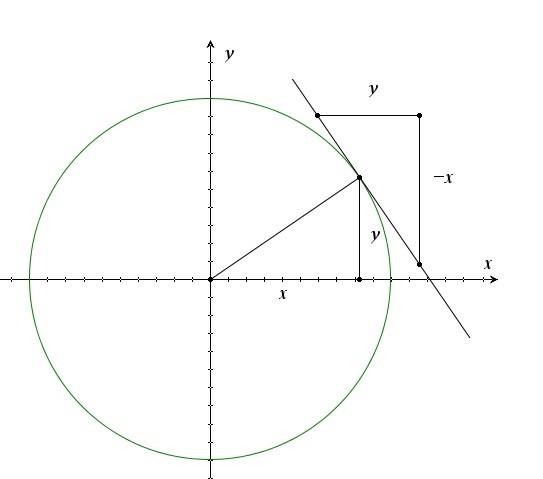# The circle and implicit derivatives

Up a level : Differentiation, derivatives
Previous page : dx/dy in three different ways
Next page : The relation y^3+y^2=x^3+x^2Let us look at the equation of a circle${x^2} + {y^2} = {r^2}$

We could of course make y the subject and take the derivative of that, but let us instead take the implicit derivative. We get$2x + 2yy' = 0$

or$y' = - \frac{x}{y}$

So what does that tell us? Let us look at a figure:We know that the radius of and the tangent to a circle at a point are perpendicular to each other, and that the slope of two perpendicular lines are the negative reciprocals of each other, i.e. if the slope of one line (say the radius) is x/y the slope of a perpendicular line, say the tangent line, is −y/x, and that’s exactly what we got.Up a level : Differentiation, derivatives
Previous page : dx/dy in three different ways
Next page : The relation y^3+y^2=x^3+x^2Last modified: Jan 20, 2018 @ 16:21